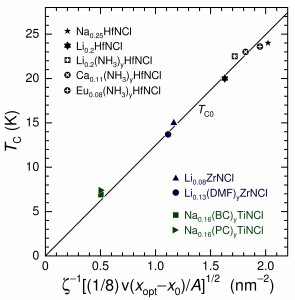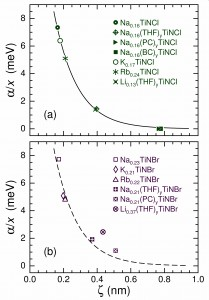# Modeling Intercalated Group-4-Metal Nitride Halide Superconductivity with Interlayer Coulomb Coupling

Modeling Intercalated Group-4-Metal Nitride Halide Superconductivity with Interlayer Coulomb Coupling, D. R. Harshman and A. T. Fiory [arXiv]
Behavior consistent with Coulomb-mediated high-TC superconductivity is shown to be present in the intercalated group-4-metal nitride halides Ax(S)yMNX, where the MNX host (M = Ti, Zr, Hf; X = Cl, Br) is partially intercalated with cations Ax and optionally molecular species (S)y in the van der Waals gap between the halide X layers, expanding the basal-plane spacing d. The optimal transition temperature is modeled by TC0 ∝ ζ–1(σ/A)1/2, where the participating fractional charge per area per formula unit σ/A and the distance ζ, given by the transverse AxX separation (ζ < d), govern the interlayer Coulomb coupling. From experiment results for β-form compounds based on Zr and Hf, in which concentrations x of Ax are varied, it is shown that σ = γ[v(xopt − x0)], where xopt is the optimal doping, x0 is the onset of superconducting behavior, v is the Ax charge state, and γ = 1/8 is a factor determined by the model. Observations of TC < TC0 in the comparatively more disordered α-Ax(S)yTiNX compounds are modeled as pair-breaking by remote Coulomb scattering from the Ax cations, which attenuates exponentially with increasing ζ. The TC0 values calculated for nine Ax(S)yMNCl compounds, shown to be optimal, agree with the measured TC to within experimental error. The model for TC0 is also found to be consistent with the absence of high-TC characteristics for AxMNX compounds in which a spatially separated intercalation layer is not formed.Plot of measured transition temperature TC versus ζ−1[(1/8) v(xopt − x0)/A]1/2 for the high-TC compounds listed in Table 1 and compounds with α = 0 from Table 2. Interaction distance ζ is from Eq. (1), v(xopt − x0) is the optimal doping xopt relative to the superconductivity onset value x0 and multiplied by valence v, and A is basal area per formula unit. The line represents TC0 for optimal compounds from Eq. (6).Pair breaking parameter α from Table 2 normalized to alkali content x and plotted against interaction distance ζ for the α-form compounds, (a) Ax(S)yTiNCl and (b) Ax(S)yTiNBr. The curves represent the function of Eq. (5) fitted to the Ax(S)yTiNCl compounds.

Dale R. Harshman and Anthony T. Fiory, J. Supercond. Nov. Mater. 28, 2967 (2015).